﻿ 改进的希尔伯特-黄变换在基于PPG信号血压测量中的应用 PPG Signal-Based Blood Pressure Estimation Using Modified Hilbert-Huang Transform

Journal of Sensor Technology and Application
Vol.06 No.02(2018), Article ID:24600,9 pages
10.12677/JSTA.2018.62008

PPG Signal-Based Blood Pressure Estimation Using Modified Hilbert-Huang Transform

Xiaohang Zhao, Fubo Deng, Yunfang Jia*

College of Electronic and Optical Engineering, Nankai University, Tianjin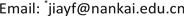Received: Apr. 7th, 2018; accepted: Apr. 20th, 2018; published: Apr. 27th, 2018ABSTRACT

A blood pressure measurement system based on photoplethysmography (PPG) signal is proposed including a hardware setup for signal collecting and a modified Hilber-Huang Transform (HHT) based PPG signal processing method. In the setup, mobile phone (MP) is used as the upper controller, under its control, PPG signal generated by a finger clip probe is collected, amplified, filtered, stored in SD card and transferred to MP. Furthermore, based on the MATLAB software, a modified HHT algorithm is proposed. Comparisons with wavelet threshold method are performed to evaluate the effectiveness of the proposed method from the views of ratio of signal to noise, feature extraction and blood pressure (BP) calculation. The experimental results indicate though the two-level Rigrsure wavelet threshold denoising method has the best performance in PPG signal denoising, the characteristic information of the processed data is also in great difference with the actual value. In contrast, the proposed modified HHT algorithm makes a little deviation, and retains the basic characteristics of the single periodic signal successfully. In conclusion, a MP based PPG portable setup and a modified HHT signal processing method are proposed and applied for BP estimation, their promising future will be in daily health inspection for the elderly, rehabilitation of patients, etc.

Keywords:PPG Signal, Wavelet Transform, Modified HHT, Blood Pressure1. 引言

2. 方法与原理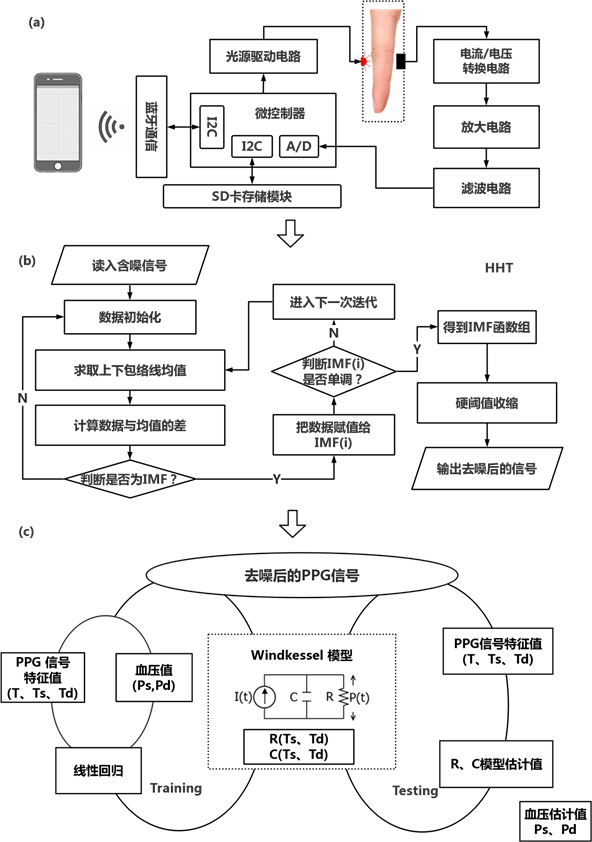Figure 1. The diagram of this work (a) hardware for PPG acquisition, (b) signal denoising process, (c) calculating method for BP estimation

2.1. 信号获取

2.2. PPG信号去噪

$DWT\left(a,b\right)={\sum }_{n=-\infty }^{+\infty }x\left(n\right){\psi }_{a,b}\left(n\right)$ (1)

${\psi }_{a,b}\left(n\right)={2}^{-\frac{a}{2}}\psi \left({2}^{-a}n-b\right)$ (2)

$x\left(t\right)=\sum _{i=1}^{N}{h}^{\left(i\right)}\left(t\right)+d\left(t\right)$ (3)

${y}_{j}\left(t\right)=\frac{P}{\text{π}}\underset{-\infty }{\overset{+\infty }{\int }}\frac{{h}_{j}\left(\tau \right)}{t-\tau }\text{d}\tau$ (4)

${z}_{j}\left(t\right)={h}_{j}\left(t\right)+i{y}_{j}\left(t\right)={a}_{j}\left(t\right){\text{e}}^{i\phi \left(t\right)}$ (5)

${a}_{j}\left(t\right)={\left[{h}_{j}^{2}\left(t\right)+{y}_{j}^{2}\left(t\right)\right]}^{1/2},\text{\hspace{0.17em}}{\phi }_{j}\left(t\right)=\mathrm{arctan}\left({y}_{j}\left(t\right)/{h}_{j}\left(t\right)\right)$ (6)

${a}_{j}$${\phi }_{j}$ 分别为第j阶IMF的瞬时幅度和相位。瞬时相位 ${\phi }_{j}\left(t\right)$ 的时间导数是瞬时频率 ${\omega }_{j}\left(t\right)$ 的物理意义，如下所示：

${\omega }_{j}\left(t\right)=\frac{\text{d}{\phi }_{j}\left(t\right)}{\text{d}t}$ (7)

$s\left(t\right)=\mathrm{Re}\left[\sum _{j=1}^{n}{a}_{j}\left(t\right){\text{e}}^{i\int {w}_{j}\left(t\right)\text{d}t}\right]$ (8)

2.3. 血压估计算法

$\frac{P\left(t\right)}{R}+C\frac{\text{d}P\left(t\right)}{\text{d}t}=I\left(t\right)$ (8)

${I}_{0}=\frac{{C}_{0}{T}_{c}}{60{\int }_{0}^{{T}_{s}}\mathrm{sin}\left(\text{π}t/{T}_{s}\right)\text{d}t}$ (9)

${P}_{s}={P}_{ts}{\text{e}}^{-{T}_{s}/RC}+\frac{{I}_{0}{T}_{s}C\text{π}{R}^{2}}{{T}_{s}{}^{2}+{C}^{2}{\text{π}}^{2}{R}^{2}}\left(1+{\text{e}}^{-{T}_{s}/RC}\right)$ (10)

${P}_{d}={P}_{td}{\text{e}}^{-{T}_{d}/RC}$ (11)

3. 结果与讨论

3.1. 光源驱动电压选择

3.2. 去噪效果

WT方法中，wden函数可选择不同的分解层数、阈值函数以及阈值处理过程。该函数可提供前文提到的四种阈值方法(Heursure、Sqtwolog、Minimax、Rigrsure)。分别比较四种不同阈值选择下小波阈值去噪算法的表现，并纵向讨论不同分解层数对于阈值去噪的影响，同时与改进的HHT去噪算法做了比较。由于脉搏波信号特征主要分布在0~10 Hz，故而仅对2~4层分解系数进行讨论。

$\text{PSNR}=10{\mathrm{log}}_{10}\left(\frac{{x}_{\mathrm{max}}^{2}}{\text{MSE}}\right)$ (12)

$\text{MSE}=\frac{1}{N}{\sum }_{t=1}^{N}{\left[x\left(t\right)-s\left(t\right)\right]}^{2}$ (13)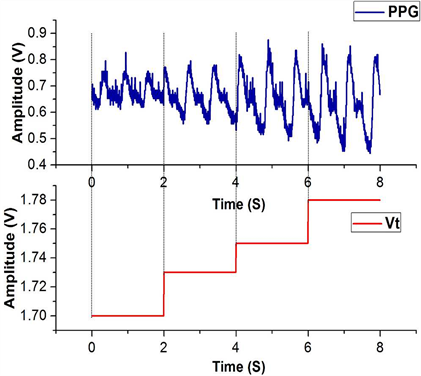Figure 2. PPG signals in different voltages

3.3. 血压估算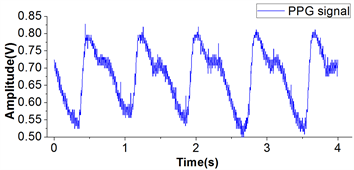(a)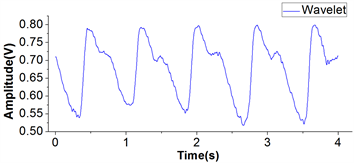(b)(c)(d)

Figure 3. (a) PPG signal detected by the system, (b) Signal after Wavelet denoising, (c) Signal after modified HHT denoising, (d) Comparison of PSNR values for different algorithms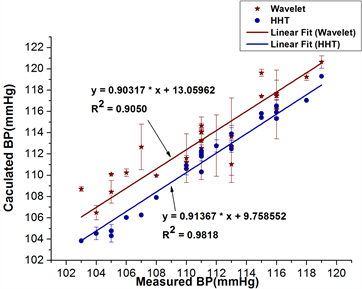(a)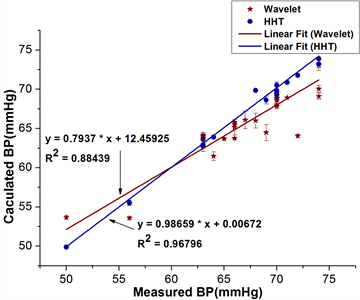(b)

Figure 4. BP values comparison of the two test methods: (a) Systolic blood pressure (Ps), (b) Diastolic blood pressure (Pd)

4. 结论

PPG Signal-Based Blood Pressure Estimation Using Modified Hilbert-Huang Transform[J]. 传感器技术与应用, 2018, 06(02): 68-76. https://doi.org/10.12677/JSTA.2018.62008

1. 1. Ahlstrom, C., Johansson, A., Uhlin, F., Länne, T. and Ask, P. (2005) Noninvasive Investigation of Blood Pressure Changes Using the Pulse Wave Transit Time: A Novel Approach in the Monitoring of Hemodialysis Patients. Journal of Artificial Organs, 8, 192-197. https://doi.org/10.1007/s10047-005-0301-4

2. 2. Choi, Y., Zhang, Q. and Ko, S. (2013) Noninvasive Cuffless Blood Pressure Estimation Using Pulse Transit Time and Hilbert-Huang Transform. Computers & Electrical Engineering, 39, 103-111. https://doi.org/10.1016/j.compeleceng.2012.09.005

3. 3. Teng, X.F. and Zhang, Y.T. (2016) An Evaluation of a PTT-Based Method for Noninvasive and Cuffless Estimation of Arterial Blood Pressure. 28th Annual International Conference of the IEEE En-gineering in Medicine and Biology Society, 30 August-3 September 2006, New York, NY, 6049-6052.

4. 4. Mccombie, D.B., Reisner, A.T. and Asada, H.H. (2006) Adaptive Blood Pressure Estimation from Wearable PPG Sensors Using Peripheral Artery Pulse Wave Velocity Measurements and Multi-Channel Blind Identification of Local Arterial Dynamics. 28th Annual International Conference of the IEEE Engineering in Medicine & Biology Society, 30 August-3 September 2006, New York, NY, 3521. https://doi.org/10.1109/IEMBS.2006.260590

5. 5. Westerhof, N., Lankhaar, J.W. and Westerhof, B.E. (2009) The Arterial Windkessel. Medical & Biological Engineering & Computing, 47, 131-141. https://doi.org/10.1007/s11517-008-0359-2

6. 6. Choudhury, A.D., Banerjee, R., Sinha, A. and Kundu, S. (2014) Estimating Blood Pressure Using Windkessel Model on Photoplethysmogram. 2014 36th Annual International Conference of the IEEE Engi-neering in Medicine and Biology Society, 26-30 August 2014, Chicago, IL, 4567-4570. https://doi.org/10.1109/EMBC.2014.6944640

7. 7. Brouse, C.J., Gatzke, R., Freeman, D. and Chen, Y. (2014) Increasing the Dynamic Range of a Pulse Oximeter Using Heart Rate Characteristics. Computing in Cardiology Conference, 41, 793-796.

8. 8. Kasambe, P.V. and Rathod, S.S. (2015) VLSI Wavelet Based Denoising of PPG Signal. Procedia Computer Science, 49, 282-288. https://doi.org/10.1016/j.procs.2015.04.254

9. 9. Joseph, G., Joseph, A., Titus, G., Thomas, R.M. and Jose, D. (2014) Photoplethysmogram (PPG) Signal Analysis and Wavelet De-Noising. 2014 Annual International Conference on Emerging Research Areas: Magnetics, Machines and Drives, 1-5. https://doi.org/10.1109/AICERA.2014.6908199

10. 10. Bai, T., Li, D., Wang, H.Q., Pang, Y., Li, G.Q., Lin, J.Z., Zhou, Q.N. and Jeon, G. (2017) A PPG Signal De-Noising Method Based on the DTCWT and the Morphological Filtering. IEEE International Conference on Signal-Image Technology & Internet-Based Systems, 24-26 July 2014, Kottayam, 503-506.

11. 11. Norden, E.H., Zheng, S., Steven, R.L., Manli, C.W., Hsing, H.S., Zheng, Q.N., Yen, N.C., Chi, C.T. and Henry, H.L. (1998) The Empirical Mode Decomposition and the Hilbert Spectrum for Nonlinear and Non-Stationary Time Series Analysis. Proceedings of the Royal Society of London A: Mathematical, Physical and Engineering Sciences, 454, 903-995.

12. 12. Tang, J., Zou, Q, Tang, Y., Liu, B. and Zhang, X.K. (2007) Hilbert-Huang Transform for ECG De-Noising. IEEE The 1st International Conference on Bioinformatics and Biomedical Engineering, 6-8 July 2007, Wuhan, 664-667. https://doi.org/10.1109/ICBBE.2007.173

13. 13. Han, G., Lin, B. and Xu, Z. (2017) Electrocardiogram Signal Denoising Based on Empirical Mode Decomposition Technique: An Overview. Journal of Instrumentation, 12, P03010. https://doi.org/10.1088/1748-0221/12/03/P03010

14. 14. Zhang, Y., Gao, Y., Wang, L., Wang, L., Chen, J.H. and Shi, X.L. (2007) The Removal of Wall Components in Doppler Ultrasound Signals by Using the Empirical Mode Decomposition Algorithm. IEEE Transactions on Biomedical Engineering, 54, 1631-1642. https://doi.org/10.1109/TBME.2007.891936

15. 15. Bi, N., Sun, Q., Huang, D., Yang, Z.H. and Huang, J.W. (2007) Robust Image Watermarking Based on Multiband Wavelets and Empirical Mode Decomposition. IEEE Transactions on Image Processing, 16, 1956-1966. https://doi.org/10.1109/TIP.2007.901206

16. 16. Molla, M.K.I. and Hirose, K. (2007) Single-Mixture Audio Source Separation by Subspace Decomposition of Hilbert Spectrum. IEEE Transactions on Audio, Speech, and Language Processing, 15, 893-900. https://doi.org/10.1109/TASL.2006.885254

17. 17. Singh, B.N. and Tiwari, A.K. (2006) Optimal Selection of Wavelet Basis Function Applied to ECG Signal Denoising. Digital Signal Processing, 16, 275-287. https://doi.org/10.1016/j.dsp.2005.12.003

NOTES

*通讯作者。